# Lesson 10: More Subtle Population Models

Population models are not limited to births and deaths. All kinds of quantifiable things pass through populations, from income to diseases. In this lesson, we will look at the transfer of information through a population, in the form of a rumor

As always, we need to be explicit about our assumptions. We'll assume the following:

1. Either you have heard the rumor (are "informed"), or you have not (are "ignorant").
2. Nobody is both informed and ignorant.
3. Once you're informed, you stay informed -- no forgetting.
4. The total population is constant over time.
5. The rumor spreads in only one way: by interactions between ignorant and informed. Interactions between informed and informed, or between ignorant and ignorant, do nothing to affect the spread of the rumor.
6. Interactions between people are random, and not made more or less likely according to whether the people in question are ignorant or informed.

# The Mathematical Model

Each of these assumptions could, in fact, be called into question. In particular, we've left no room in our model for someone who hears, but does not believe enough to pass on, the rumor. Perhaps assumption 6 is particularly shaky. But let's see if we can translate assumptions 1-6 into mathematical equations, to see what they predict about the spread of the rumor. Then we can come back and refine the model as needed.

a video walkthrough of this

Assumptions 1-3 all come together to say that the rumor spreads by converting people from ignorant to informed. Assumption 4 says there's no new ignorant people being created, nor are there informed people dying or otherwise leaving the community. So we haveIt will be convenient to work with rates of increase across the board, so let's restate that as: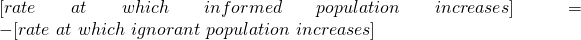Now, assumption 5 says: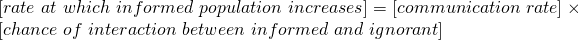where by communication rate I mean the fraction of the time that, during an interaction between an informed person an ignorant person, the rumor is communicated in that interaction.

Now we need to count the chance of interaction between an informed and an uninformed person. But this is straightforward: since the two events are independent, we have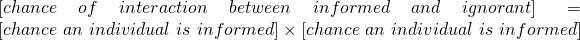Now we're ready to make everything symbolic. Use:

 symbol meaning t time (hours) P(t) ignorant population Q(t) informed population N total population r communication rate

The chance of picking an ignorant individual is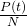; the chance of picking an informed individual is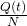.

We have: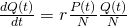And since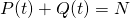, we can write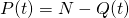and substitute to obtain the differential equation: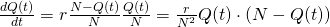Actually let's clean this up a bit. We could write it in the following way: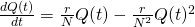where the first term looks like the population growth model that gave us the exponential function, and the second term (which has a negative sign in front) counteracts that explosive growth.

### Some A Priori Reasoning

Let's see what the equation itself tells us. First, what happens when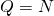, that is, when everyone is informed? Then the right-hand side of our equation is 0, so we have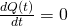. That is, nobody is becoming informed anymore. That makes sense -- there's nobody to become informed if everyone already knows. What about when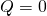, that is, if nobody knows the rumor? Then again, the right-hand side is 0, so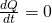; nobody becomes informed. But if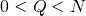, then the right-hand side is positive, which means people are learning about the rumor. That seems reasonable.

We call any level of the population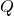for whichan equilibrium or steady state of the model. They are called this, because whenreaches one of these levels, it just stays there and never changes. We can find equilibria by solving the algebraic equation obtained by setting the right-hand side equal to zero. In this case, we'd need to solve: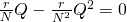(by "solve", I mean solve for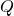.) We could do this by hand, or have Maple do it for us:(So we see that in fact,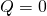(nobody knows the rumor) and(everybody knows the rumor) are the only equilibria.

### Solving the Equation (Maple)

Let's get Maple to solve the equation for us.

a video walkthrough of this

1. Enter the equation -- call it "rumormodel", because that's what it is.2. Use the dsolve command to solve the initial value problem with the initial value Q0:Since that's fairly ugly (and anyway, inscrutable), let's try to plot it. To plot, we need to specific values for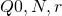. Let's try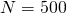(500 people in the community),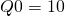(10 of them initially know the rumor),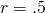(half the time an ignorant person runs into an informed person, the rumor is transmitted). We carry this out using subs:Now let's plot that to see what we get. particularsolution is an equation, but what we want to plot is a function. So we tell Maple we want to just plot the right-hand side of particularsolution:That's the first 1000 hours (that's a bit less than 42 days). Looks almost linear, but actually it's closer to exponential. At this point, looks like we're up to about 26 people who know of the rumor. Out of the community of 500, that's not so many. After 2000 hours (83 days and change):the rumor is up to about 65 informed people, and the spread is really starting to look exponential. The function we found isn't exactly exponential, though, so let's keep going to see what's up. After 5000 hours (208 days, almost seven months), the rumor has spread so that well over half the town knows about it.Notice that now, the rumor is still spreading, but actually its spread has started to slow just a bit. (That's in contrast to the exponential growth, which is always speeding up.

After a year (8760 hours), the population which knows about the rumor is almost up to 500 (the entire community). We can see that the rate of spread has recently dropped off quite a bit:### Visualizing Multiple Solutions (Maple)

In addition to solving and then plotting a particular solution, we can get Maple to draw us a picture that indicates what all solutions look like. We'll need to load a library for this.That's a lot of tools! We want to use one called DEplot, which requires us to have no parameters in our differential equation. So first we use subs to plug in some values:Then we invoke DEplot:The DEplot command

[>DEplot(particularmodel,Q(t),t=0..1000,[Q(0)=10])

tells Maple to plot the solution to our model, for the first 1000 hours, starting with the initial condition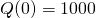. The curve in yellow is our solution. The red arrows nearby are called a slope field; they indicate the direction other solutions ought to follow. Let's try changing our time range, say to cover the first 8000 hours. We'll also ask Maple to plot what would happen if the initial group of people who knew the rumor had been 100 and 500 people:DEplot gives us a way to visualize and understand the model as a whole, rather than just particular solutions. Notice that the solutions (yellow curves) follow along with the red arrows.

# Some other populations

### The Logistic Model

Let's try to make our population growth model from Lesson 8 a little more realistic. To obtain that model, we assumed that each bacterium would be reproducing at a rate that didn't depend on anything. But in reality, once you get a bunch of bacteria, they'll start competing with one another for food and space. That competition slows down reproduction. We have the word equation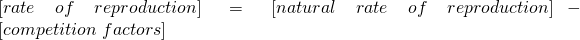Competitive encounters require two bacteria (namely, the ones that are interacting). Every time two bacteria interact, there some chance (call it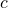) that something untoward happens.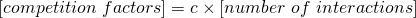Now if we use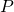to represent the bacterial population, the number of interactions should be some multiple of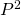: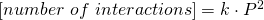As in Lesson 8, the natural reproduction rate is: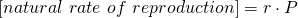All together, we have: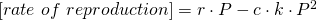If we set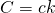, then we've got the differential equationwhich could also be written as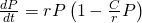What are the equilibria? Let's get Maple to tell us. The equation we are dealing with is called the logistic equation, so we'll have Maple refer to it as that. Remember that the equilibria of a differential equation are when the right-hand side is zero. So we just kindly ask Maple to solve for us:There are two equilibria: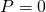(no bacteria), andthe so-called carrying capacity, which represents the maximum supportable population of bacteria that can be supported given the constants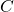and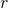. For this reason, we often rewrite the logistic equation aswhere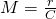.

### Logistic Model with Harvesting

Now let's say that we continuously remove some of the bacteria, say by installing a little drain in the dish where they grow and suctioning them out at a constant rate (this is referred to as "harvesting"). We'd need to update our word equation to:Harvesting is simple: we just remove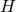bacteria per hour from the population. So the differential equation becomes: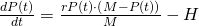Use Maple to find the equilibria for the logistic model with harvesting. You'll notice that, unlike the exponential model and the logistic model,is not an equilibrium for this model!

# Takeaways/Deliverables

To say you've successfully completed this lesson, you should be able to do the following:

1. Use Maple's solve command to find equilibria of differential equations.
2. Use Maple's dsolve command to find explicit solutions to initial value problems.
3. Use DEplot to graph slope fields and solutions of initial value problems.

These skills are what you'll need to complete the WebAssign homework for this Lesson.